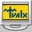Math Center Level 11.0.1.9

Software Information
• 0 / from 0 votes
• Views : 487
• File Size : 1.9 MB
•1/57 Virus Flags
From: Tvalx
Math software for students studying precalculus. Can be interesting for teachers teaching precalculus. Math Center Level 1 consists of Graphing calculator 2D Advanced Calculator Simple Calculator Simple Calculator Simple Rational Calculator and Simple Integer Calculator called from the Control Panel. Simple calculator is a general purpose calculator which combines use simplicity and calculation power. It handles simple arithmetic operations and complex formulas. Advanced Calculator is a step farther in complexity comparing to the Simple Calculator. The Advanced Calculator has two editing windows. One is for editing x and the second is for editing f(x). In the x window you can enter any number or formula which contains numbers. In the f(x) window you can enter formulas containing numbers and formulas containing x. First x will be calculated. Then the result for x will be substituted into the formula for f(x). The presence of two editing windows demands switching between windows. You can do it by clicking buttons go to x and go to f(x) or by clicking inside the window. If you forget to enter x then the x1 will be assumed. If you forget to enter f(x) then f(x)x will be assumed. Advanced Calculator works in scientific mode. All numbers in internal calculations are treated in scientific format. Graphing Calculator 2D has two panels. The Left Panel has the Magnifying Square represented by Small Square with gray border on the Left Panel. It is 16 times smaller than the Left Panel. The Right Panel shows content of the Magnifying Square magnified 16 times. You can press button zoom +. Then the Left and Right Panels will be zoomed twice each. Maximum zoom is 8 (tree clicks of zoom +). Clicking button C (for Center) on Zoom returns picture to starting position with no zoom and Magnifying Square at the center of Left Panel.

 Title Math Center Level 1 Author Tvalx File Name MathCenterLevel1.exe File Size 1.9 MB OS Win2000,Win7 x32,Win7 x64,Win98,WinServer,WinVista,WinVista x64,WinXP Languages English Published On 2015-02-06 09:54:06 Change Log improvement Tagged As

Free Alternatives

•Math Games Level 1
•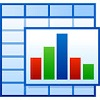MedCalc
•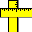Breaktru Quick Conversion
•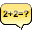Calculator Prompter
•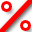Breaktru Percent

User Reviews

0 Reviews, be the first to review this product.Cyclance is a smart antivirus that uses artificial intelligence to keep your system fully protected

2018-08-24 07:42:42

Software Information
• 0 / from 0 votes
•1/57 Virus Flags